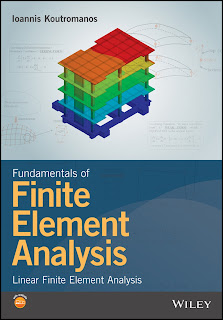DISCRETE MATHEMATICS

# Fundamentals of Finite Element Analysis Linear Finite Element Analysis PDF Free DownloadThis book constitutes the first volume in a two-volume set that introduces readers to the theoretical foundations and the implementation of the finite element method (FEM). The first volume focuses on the use of the method for linear problems. A general procedure is presented for the finite element analysis (FEA) of a physical problem, where the goal is to specify the values of a field function. First, the strong form of the problem (governing differential equations and boundary conditions) is formulated. Subsequently, a weak form of the governing equations is established. Finally, a finite element approximation is introduced, transforming the weak form into a system of equations where the only unknowns are nodal values of the field function. The procedure is applied to one-dimensional elasticity and heat conduction, multi-dimensional steady-state scalar field problems (heat conduction, chemical diffusion, flow in porous media), multi-dimensional elasticity and structural mechanics (beams/shells), as well as time-dependent (dynamic) scalar field problems, elastodynamics and structural dynamics. Important concepts for finite element computations, such as isoparametric elements for multi-dimensional analysis and Gaussian quadrature for numerical evaluation of integrals, are presented and explained.